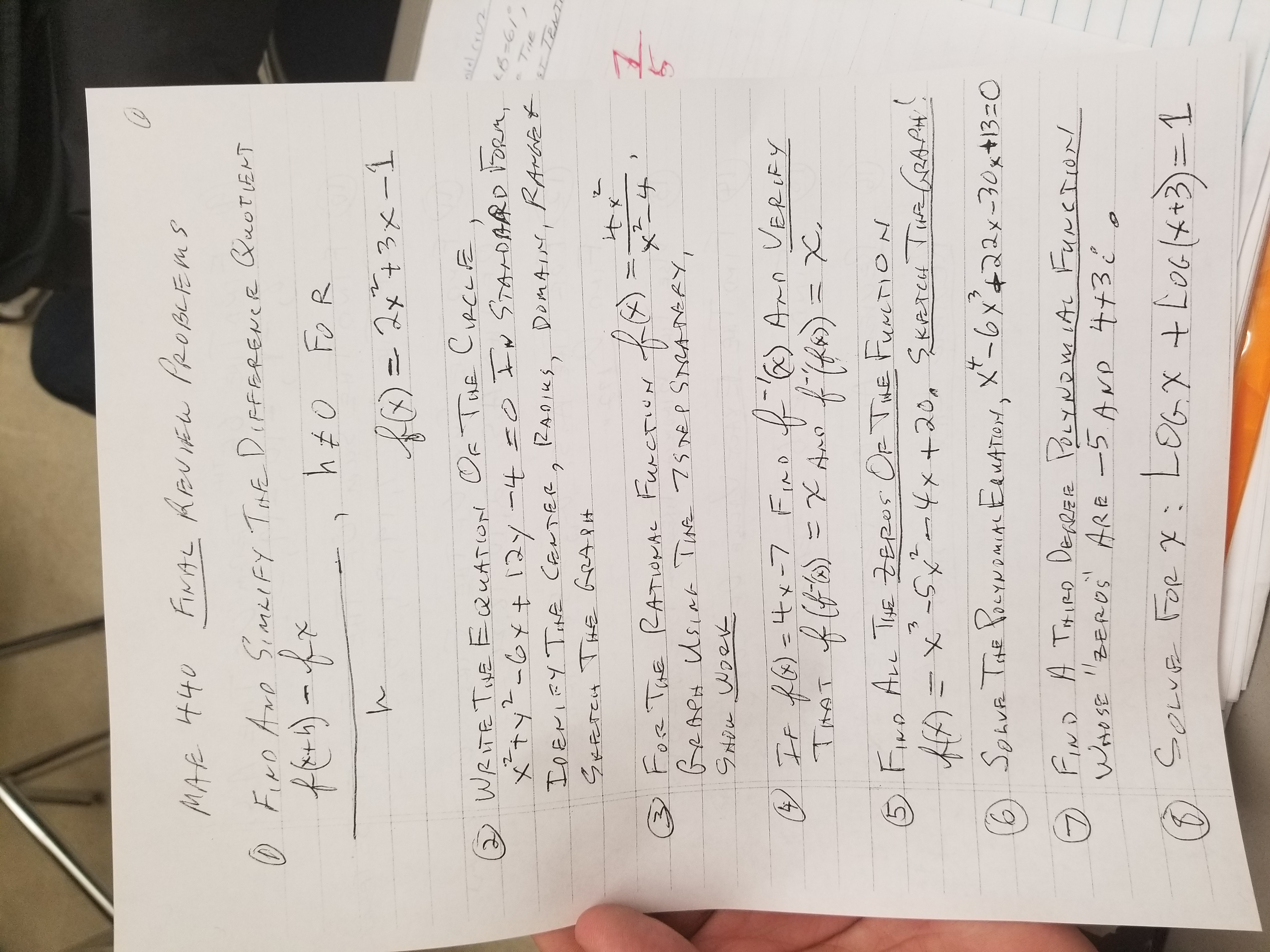# FINAL REUIEW PROBLEMSMAR 440QUOTENTO Firo AroSimeIFy Tfe DifFEREweRh £o Fo R2x73x-1f0)=WRITE THE EQUATION Of Tue CircLExtyt-Gy+ 12y -4 =0 In STADARD ORM,IDENIFY Teme CENTERR2CRCLFEniel cruB=61RAMafet,IDeniFy Tne CanTER, RADIKS,SKFETCH TH€ GRAPHDOMAIN(ZANIKSTitKo)3Fose TuE RATIONAL FurcTIUN5BeAeH Usiar Tiae 7sse p SreAnERy,f6) Arn VERLEY) If RG)=4x-7 Fino f G) Arn=X Amo ff) = X(Rs)fiR))3Dx,TimAT f)5 Fue ALL TiHE ZEROS CF TME FuncTION-4x+20, Skereu Tue CRALA!HA=xitX'-6x422x-30x+13=06 SonvR The PoeYNaMIAL EauATON,NDMIALFIND A THIRD DEARER OLYNOW IAL FUNCTION!Woto SE "ZEROS ARE -5 AND 4+3 ¿ oLeO Sowe FOR x: LOG.X + LoGl+3)=1

Question
2 viewshelp_outlineImage TranscriptioncloseFINAL REUIEW PROBLEMS MAR 440 QUOTENT O Firo Aro SimeIFy Tfe DifFEREweR h £o Fo R 2x73x-1 f0)= WRITE THE EQUATION Of Tue CircLE xtyt-Gy+ 12y -4 =0 In STADARD ORM, IDENIFY Teme CENTERR2 CRCLFE niel cru B=61 RAMafet , IDeniFy Tne CanTER, RADIKS, SKFETCH TH€ GRAPH DOMAIN (ZANIKS Tit Ko) 3 Fose TuE RATIONAL FurcTIUN 5 BeAeH Usiar Tiae 7sse p SreAnERy, f6) Arn VERLEY ) If RG)=4x-7 Fino f G) Arn =X Amo ff) = X (Rs) fiR))3Dx, TimAT f) 5 Fue ALL TiHE ZEROS CF TME FuncTION -4x+20, Skereu Tue CRALA! HA=x it X'-6x422x-30x+13=0 6 SonvR The PoeYNaMIAL EauATON, NDMIAL FIND A THIRD DEARER OLYNOW IAL FUNCTION! Woto SE "ZEROS ARE -5 AND 4+3 ¿ o Le O Sowe FOR x: L OG.X + LoGl+3)=1 fullscreen
check_circle

Step 1

Rearrange the terms, so that x terms are together and y terms are together. Add 4.

Step 2

Complete square for x and y terms separately.

Answer: Standard form: (x-3)^2 + (y+6)^2 = 49

Step 3

Compare the equation with (x-h)^2+(y-k)^2...

### Want to see the full answer?

See Solution

#### Want to see this answer and more?

Solutions are written by subject experts who are available 24/7. Questions are typically answered within 1 hour.*

See Solution
*Response times may vary by subject and question.
Tagged in

### Calculus